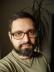## The Encoder

Write a program to encode text into binary encoded Morse code.

Before we can encode Morse code into its binary representation, we need to encode normal text into Morse code. As a former Woodcraft member, I was able to write the following lines by heart:

``````my %to_morse = qw( a .-   b -... c -.-. d -..  e .    f ..-. g --.
h .... i ..   j .--- k -.-  l .-.. m --   n -.
o ---  p .--. q --.- r .-.  s ...  t -    u ..-
v ...- w .--  x -..- y -.-- z --.. );``````

The encoding subroutine is straightforward: split each word into separate characters, then replace each with the value from the above hash.

``````sub encode_to_morse {
join '/', map \$to_morse{\$_} // "", split //, shift
}``````

Note that space is not present in the translation table, so it gets translated to an empty string, which creates the expected double slashes between words.

The next step is to decode Morse code into its binary form. It’s defined in the following way:

 dot 1 dash 111 intra-character gap 0 character gap 000 word gap 0000000

The zeros and ones represent bits, but for simplicity, we’ll start with normal digits.

``````use Syntax::Construct qw{ /r };

my %to_binary = ('.'  => 1,
'-'  => 111,
""   => 0,
'/'  => '000',
'//' => '0000000');

sub encode_to_binary_digits {
encode_to_morse(shift) =~ s,( (?<=[-.]) (?=[-.]) | //? | [-.] )
,\$to_binary{\$1},grx
}``````

There are several tricks that need explanation. We’re replacing any occurrence of a dot, dash, slash, or two slashes with the corresponding code from the translation table. But what does the first element in the alternative match? It says “preceding symbol is a dash or dot” and “the following symbol is a dash or dot”, which in fact matches an empty string inside a letter. That’s why we have an empty string in our translation table. The `?` quantifier is greedy, so `//` can never generate two matches of a single slash.

We now need to convert the binary digits to actual bits. Perl already knows how to do that: we can use the `b` template of pack.

``````sub encode_to_binary {
pack 'b*', encode_to_binary_digits(shift)
}``````

The asterisk means there can be any number of bits. The result is padded to fit into bytes, which we’ll have to remember to round-trip the result.

## The Decoder

Write a program to decode binary Morse code.

We’ll again proceed in steps similarly to the encoder, but in the opposite direction. The input is a padded binary string, so we just need to remove the padding zeros after applying unpack with the template we’ve already seen.

``````sub decode_to_binary_digits {
unpack('b*', shift) =~ s/0*\$//r
}``````

To get Morse code from the binary digits, we need to reverse the translation table.

``````my %from_binary = reverse %to_binary;
sub decode_to_morse {
join "",
map \$from_binary{\$_},
split /(0+)/,
decode_to_binary_digits(shift)
}``````

We’re splitting the digits on sequences of zeros, but by creating a capture group in split, the zeros will be returned by `split`, as well, so they’ll be correctly translated to nothing, a slash, or two slashes.

To decode the resulting Morse code, we’ll use the first translation table reversed.

``````my %from_morse = ('/' => "", '//' => ' ', reverse %to_morse);

sub decode_from_binary {
join "", map \$from_morse{\$_}, split m{(/+)}, decode_to_morse(shift)
}``````

We added slashes to the table to correctly insert spaces into the output text. Again, we’re using a capture group in split, so the slashes are getting translated, too.

To verify the round-trip, I encoded a short string by hand and wrote the following tests:

``````use Test::More tests => 6;

my \$input = 'just another';
my \$morse = '.---/..-/.../-//.-/-./---/-/...././.-.';
my \$digits = '101110111011100010101110001010100011100000001011100'
. '01110100011101110111000111000101010100010001011101';
my \$bin    = join "", map chr,
221, 29, 117, 84, 28, 208, 113, 113, 119, 28, 85, 68, 23;

is encode_to_morse(\$input), \$morse, 'encode to morse';

is encode_to_binary_digits(\$input), \$digits, 'encode to binary digits';

is encode_to_binary(\$input), \$bin, 'encode to binary';

is decode_to_binary_digits(\$bin), \$digits, 'decode to binary digits';

is decode_to_morse(\$bin), \$morse, 'decode to morse';

is decode_from_binary(\$bin), \$input, 'decode from binary';``````I blog about Perl.